# Reports

Surfer can generate several types of detailed grid reports. This table provides a snapshot of the types of information produced for each report type. Descriptions of the report sections are below the table.

If you make changes to the data selection (i.e. changing a data column or changing the data filtering method) generate a new report by repeating one of the processes listed with the report type.

 Information Produced Report Types and How to Access Them Cross Validation Report Data Statistics Report Grid Contour Vol/Area Report Gridding Report Grid Information Report Variogram Data Report Click Report in the Cross Validation dialog Click the Statistics button in the Grid Data dialog Click the Grids | Calculate | Contour Vol/Area command Check the Grid Report Option on the Grid Data dialog Click the Grids | Info | Grid Info command; or click Options | Grid Info in the Grid Editor In the Variogram Properties click the Display Statistics button on the Statistics page and then click Report Data Counts, includes Filtered and at Validation Points X X X X Filtering, includes Breakline, Duplicate, Exclusion X X Grid Information, includes Grid Geometry X X Gridding Rules X X Inter-Variable Correlation, Rank Correlation and Covariance X X X X Output Grid X Principal Component Analysis X X Polygon Used for Statistics X Regression, includes Planar: Z=AX+BY+C and Residual: R=AX+BY +C X X X Statistics, such as Univariate, Nearest Neighbor, and Cross-Validation X X X X X Variogram Grid X Volume and Area X Z Transform, includes Grid and Data X

## Information Contained in Each Report Section

Each section of the report contains information about the grid, data, or variogram.

### Time Stamp

 Time of report Date and time the report was created in Mon Oct 14 10:43:13 2013 format Elapsed time to create grid Seconds required when gridding. Only included in the Gridding Report.

### Data Source

 Source Data File Name path and file name of the data used in gridding X Column X data column Y Column Y data column Z Column Z data column Detrending variogram data detrending method selected on the General page in the New Variogram dialog. Only included in the Variogram Grid Report.

### Data Counts and Filtered Data Counts

 Active Data number of data after applying filters Original Data number of original data points (excludes breakline data) Excluded Data number of data excluded by the Data Exclusion Filter - the excluded data are listed in the Exclusion Filtering section Deleted Duplicates number of duplicates deleted by the Duplicate Data filter - the deleted duplicates are listed in the Duplicate Filtering section Retained Duplicates number of duplicates retained by the Duplicate Data filter (this statistic is not computed if the duplicate rule is ALL) - the retained duplicates are listed in the Duplicate Filtering section including any artificial data Artificial Data number of artificial data created by the Sum, Average, and Midrange Duplicate Data filters Superseded Data Superseded data are number of data eliminated by breaklines in the Data Statistics Report and the Gridding Report. Breakline data always supersede point data. If point data are on, or in the immediate vicinity of, breakline data the point data are eliminated.

### Data Counts at Validation Points

The Data Counts at Validation Points section is only included in the Cross Validation Report.

 Active Results locations at which the cross validation interpolation was successfully carried out NoData Results The NoData results are the locations at which cross validation interpolation was attempted, but was not successful. For example, the natural neighbor gridding algorithm can only interpolate at locations within the convex hull of the active data. As such, an observation that lies on the convex hull of the original, complete, data set will lie outside of the convex hull of the active data when that observation is the cross validation point. Cross validation is not possible using the natural neighbor algorithm at such a point, so it is assigned the NoData value. Attempted Results reports the number of locations at which cross validation interpolation was attempted Requested Results contains the original number of random data

### Z Data Transform

Includes the transformation method (if any) applied to the Z values. Lists the data that was unable to be transformed in a table.

The rest of the report information is calculated using the active data, including any artificial data generated by duplicate filtering. Excluded, deleted, or superseded data are not included in the following calculations.

### Exclusion Filtering

 Exclusion Filter String shows the Data Exclusion Filterstring Excluded Data number of data excluded by the Data Exclusion Filter - Excluded Data Table the excluded data are listed in a table. The ID is equal to the line number in the original data file. This list is 100 data rows long by default.

### Duplicate Filtering

 Duplicate Points to Keep To Keep filter used X Duplicate Tolerance maximum X spacing of points to be considered a duplicate Y Duplicate Tolerance maximum Y spacing of points to be considered a duplicate Deleted Duplicates number of duplicates deleted by the Duplicate Data filter - the deleted duplicates are listed in the Duplicate Filtering section Retained Duplicates number of duplicates retained by the Duplicate Data filter (this statistic is not computed if the duplicate rule is ALL) - the retained duplicates are listed in the Duplicate Filtering section including any artificial data Artificial Data number of artificial data created by the Sum, Average, and Midrange Duplicate Data filters Duplicate Data Table the duplicate data table lists all of the duplicate points with X, Y, Z, ID, and Status. The ID is equal to the line number in the original data file. When the status is artificial, no ID is given since this data does not come from the original data file. The Status (Retained, Deleted, or Artificial) reports how the duplicate was handled. This list is 100 data rows long by default.

### Breakline Filtering

When breaklines are used, data that is within the X Tolerance and Y Tolerance, as set in the Filter dialog, of the breakline are deleted due to breakline data superseding original data.

 Anisotropy Angle the anisotropy angle reported for the default variogram Anisotropy Ratio the anisotropy ratio reported for the default variogram X Tolerance maximum X spacing of points to be considered a duplicate Y Tolerance maximum Y spacing of points to be considered a duplicate Superseded Data Superseded data are number of data eliminated by breaklines in the Data Statistics Report and the Gridding Report. Breakline data always supersede point data. If point data are on, or in the immediate vicinity of, breakline data the point data are eliminated. Breakline Data Table the breakline data table lists all of the superseded data points with X, Y, Z, ID, and Status. The ID is equal to the line number in the original data file. The Status (Retained, Deleted, or Artificial) reports how the duplicate was handled. This list is 100 data rows long by default.

### Inter-Variable Correlation

The Inter-Variable Correlation table shows the correlation between the X, Y, and Z variables. The Cross Validation Report also contains Estimated Z and Residual Z columns and rows. The correlations are computed with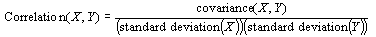The correlation is positive when both variables increase or decrease together. The correlation is negative when one variable increases while the other variable decreases. A correlation of zero shows that there is no linear relationship between the variables.

### Inter-Variable Covariance

The Inter-Variance Covariance table shows the covariance between the X, Y, and Z variables. The covariances are computed with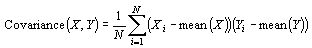The covariance is positive if, on average, the variables are both above the mean. The covariance is negative if one variable is above the mean and the other variable is below the mean.

### Inter-Variable Rank Correlation

The Inter-Variance Rank Correlation table shows the rank correlation between the X, Y, and Z variables. The data is ordered and then assigned a rank value from 1 to the count of values. Rank values range from -1 to +1. The correlation is positive when both variables increase or decrease together. The correlation is negative when one variable increases while the other variable decreases. A correlation of zero shows that there is no linear relationship between the variables.

### Univariate Statistics

This group of statistics shows information for X, Y, and Z data. These statistics do not include breakline data.

 Count total number of points 1%-tile 1 percent of the values are smaller than this number and 99 percent of the values are larger 5%-tile 5 percent of the values are smaller than this number and 95 percent of the values are larger 10%-tile 10 percent of the values are smaller than this number and 90 percent of the values are larger 25%-tile lower quartile; 25 percent of the values are smaller than this number and 75 percent of the values are larger 50%-tile middle data value, 50 percent of the data values are larger than this number and 50 percent of the data are smaller than this number 75%-tile upper quartile; 75 percent of the values are smaller than this number and 25 percent of the values are larger than this number 90%-tile 90 percent of the values are smaller than this number and 10 percent of the values are larger 95%-tile 95 percent of the values are smaller than this number and 5 percent of the values are larger 99%-tile 99 percent of the values are smaller than this number and 1 percent of the values are larger Minimum minimum value Maximum maximum value Mean arithmetic average of the data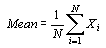Median middle data value, 50 percent of the data values are larger than this number and 50 percent of the data are smaller than this number Geometric Mean geometric mean of the data Harmonic Mean harmonic mean of the data Root Mean Square square root of the mean square Trim Mean (10%) Trim Mean is the mean without the upper five percent and lower five percent of the data, therefore, extreme value influence is removed. If there are fewer than 20 data points, the minimum and maximum data points are removed instead of the upper and lower five percent. Interquartile Mean interquartile mean, or midmean, is a truncated mean using only the data in the second and third quantiles (all data beween the 25%%-tile and 75%%-tile) Midrange the value halfway between the minimum and maximum values Midrange = (Minimum + Maximum ) / 2 Winsorized Mean Winsorized mean is a truncated mean. This method replaces the extreme highs and lows values with a more central value. This mean is less sensitive to outliers. TriMean the trimean, or Tukey's trimean, is a measure of probability distribution location. This is equivalent to the the sum of (quartile 1, 2 times the quartile 2, and quartile 3) divided by four. Variance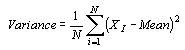Standard Deviation square root of the variance Interquartile Range separation distance between the 25%-tile and 75%-tile this shows the spread of the middle 50 percent of the data, similar to standard deviation, though this statistic is unaffected by the tails of the distribution Range separation between the minimum and maximum value Range = Maximum - Minimum Mean Difference the mean or average of the absolute difference of two random variables X and Y. Median Abs. Deviation Median Absolute Deviation is the median value of the sorted absolute deviations. It is calculated by 1. computing the data's median value 2. subtracting the median value from each data value 3. taking the absolute value of the difference 4. sorting the values 5. calculating the median of the values Average Abs Deviation Average Absolute Deviation is the average value of the sorted absolute deviations. It is calculated by 1. computing the data's average mean value 2. subtracting the mean value from each data value 3. taking the absolute value of the difference 4. calculating the average value Quartile Dispersion Measures dispersion of the data using: (Quartile 3 - Quartile 1)/(Quartile 3 + Quartile 1) Relative Mean Difference The mean difference of the entire data set divided by the sample mean of the data set Standard Error The standard error of the mean is the standard deviation of those sample means over all possible samples drawn from the population. This is calculated by dividing the standard deviation by the square root of the number of samples. Coef. of Variation The Coefficient of Variation is calculated by dividing the standard deviation by the mean. If a "-1" is reported, the coefficient of variation could not be computed. The coefficient of variation is computed only for the Z values. Skewness The Coefficient of Skewness is calculated by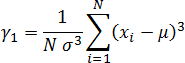If a "-1" is reported, the coefficient of skewness could not be computed. The coefficient of skewness is computed only for the Z values. Kurtosis The Coefficient of Kurtosis is calculated by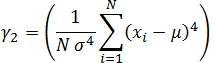Sum the sum of all X, Y, or Z values Sum Absolute the absolute value of the sum of all X, Y, or Z values Sum Squares the sum of all squared X, Y, or Z values Mean Square### Planar Regression

Planar regression is an ordinary least-squares fit where Z=AX+BY+C.

• The Parameter Values are the A, B, and C values.

• The Standard Error is the estimated standard deviation of the parameters.

• The Inter-Parameter Correlations are the correlation between A, B, and C coefficients.

• The ANOVA Table shows regression statistics on the planar fit where df are the degrees of freedom and F is the ratio of the mean squares.

• The Coefficient of Multiple Determination (R2) is calculated with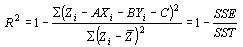For the Cross Validation Report, the planar regression is the residual regression at the validation points.

### Nearest Neighbor Statistics

The nearest neighbor statistics represent aspects of the data values and of the data locations. The nearest neighbor to a data point uses a simple separation distance without taking anisotropy into account. If two or more points tie as the nearest neighbor, the tied data points are sorted on X, then Y, then Z, and then ID. The smallest value is selected as the nearest neighbor.

The Separation column shows the separation distances between the observation and its nearest neighbor. The |Delta Z| column shows the absolute values of the differences between the observation Z value and the nearest neighbor Z value.

The statistics are the same as the Univariate Statistics (see above).

The Nearest Neighbor Statistics also includes the Complete Spatial Randomness section. The Complete Spatial Randomness statistics measure how random locations are in space. Surfer does not correct for edge effects so the statistics may be biased.

 Lamda is the average spatial density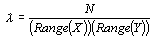Clark and Evans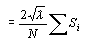where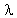= average spatial density Si= separation distance between the observation and the nearest neighbor The distribution of this statistic is normal, with a mean equal to one and a variance of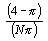See Clark and Evans (1954) and Cressie (1991) for more information. Skellam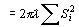where= average spatial density Si= separation distance between the observation and the nearest neighbor The distribution is Chi-Squared with 2N degrees of freedom. See Skellam (1952) and Cressie (1991) for more information.

### Principal Component Analysis

Principal component analysis (PCA) is a mathematical procedure that uses orthogonal transformation to convert a set of observations of possibly correlated variables into a set of values of linearly uncorrelated variables called principal components. The number of principal components is less than or equal to the number of original variables. The principal components are calculated for the X, Y, and Z values. Avalue is also reported for each principal component.

### Variogram Grid

 Max Lag Distance Max Lag Distance set on the General page in the New Variogram dialog Angular Divisions number of Angular Divisions set on the General page in the New Variogram dialog Radial Divisions number of Radial Divisions set on the General page in the New Variogram dialog

### Output Grid

 Grid File Name name of the output grid file Grid Size number of rows and columns in the grid Total Nodes number of columns times the number of rows Filled Nodes number of grid nodes containing interpolated values NoData Nodes number of grid nodes containing the NoData value NoData Value reports the Z value associated with NoData nodes X Minimum minimum X grid line value specified in the Output Grid Geometry group in the Grid Data dialog X Maximum maximum X grid line value specified in the Output Grid Geometry group in the Grid Data dialog X Spacing X spacing set in the Grid Data dialog Y Minimum Minimum Y grid line value specified in the Output Grid Geometry group in the Grid Data dialog Y Maximum Maximum Y grid line value specified in the Output Grid Geometry group in the Grid Data dialog Y Spacing Y spacing set in the Grid Data dialog

### Grid Information

 Grid File Name name of the output grid file Grid Size number of rows and columns in the grid Total Nodes number of columns times the number of rows Filled Nodes number of grid nodes containing interpolated values NoData Nodes number of grid nodes containing the NoData value NoData Value reports the Z value associated with NoData nodes

### Grid Geometry

 X Minimum minimum X grid line value specified in the Output Grid Geometry group in the Grid Data dialog X Maximum maximum X grid line value specified in the Output Grid Geometry group in the Grid Data dialog X Spacing X spacing set in the Grid Data dialog Y Minimum Minimum Y grid line value specified in the Output Grid Geometry group in the Grid Data dialog Y Maximum Maximum Y grid line value specified in the Output Grid Geometry group in the Grid Data dialog Y Spacing Y spacing set in the Grid Data dialog

### Polygon used for statistics

 Side Whether statistics were calculated for values Inside or Outside the polygon(s) Polygons Number of polygons used for calculating statistics Number of points Total number of vertices among the polygon(s)

### Gridding Rules

This section displays the gridding method used, as well as the option settings for each gridding method.

### Univariate Grid Statistics

The Univariate Grid Statistics are the same as those reported in the Univariate Statistics and Nearest Neighbor Statistics sections.

### Univariate Cross-Validation Statistics

The Univariate Cross Validation Statistics section are the same as those reported in the Univariate Statistics. It also contains an additional column of data, called Data Used. This column shows the number of data points used in the calculation.

### Volumes and Areas

Volume and area calculations are based on the Simple level method for contour line properties. Minimum and maximum contours along with the contour interval must be specified. Surface area and planar area calculations are made above, below, and between contours. Contour volume calculations are made above, below, and between contours.